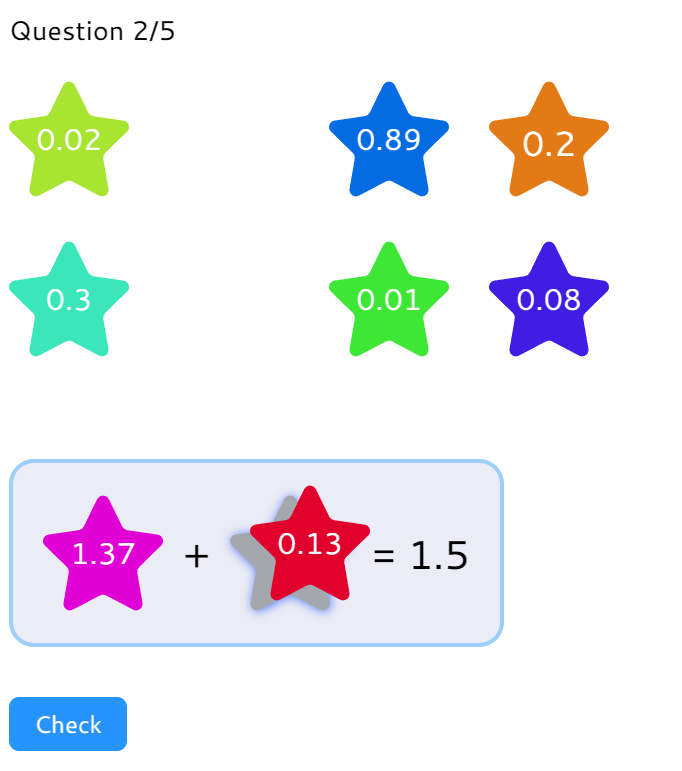MM Practice
×
Multiplication
Division
Place Value
Fractions & Decimals
Measurement
Statistics & Probability
Pre-Algebra
Money
Kindergarten
Geometry

## Make Decimal Number Sentences

#### Online practice for grades 5-7

Standard CC.5.NBT.B.7

Find two decimals and drag them to the correct places, to make addition, subtraction, multiplication, and division sentences using mental math.

You can choose the maximum number of decimal digits, to make the practice exercises easier or harder. The addition and subtraction exercises help you understand decimal place value.

The multiplication exercises practice the basics of decimal multiplication: that the product has as many decimal digits as the factors have in total.

The division exercises are based on mental math and decimal multiplication. For example, you might have 0.06 ÷ 3 = 0.02 which is a "sharing division" that you can do with mental math, or you might get 0.048 ÷ 0.006 = 8 which can be solved thinking, "How many times does the divisor fit into the dividend?"

Both types of divisions can also be solved by thinking backwards of multiplication.

Note: If you are using a phone, the game works best if you turn your phone to landscape mode.

Type:

Screenshot from the game:Question 1/5
+
=